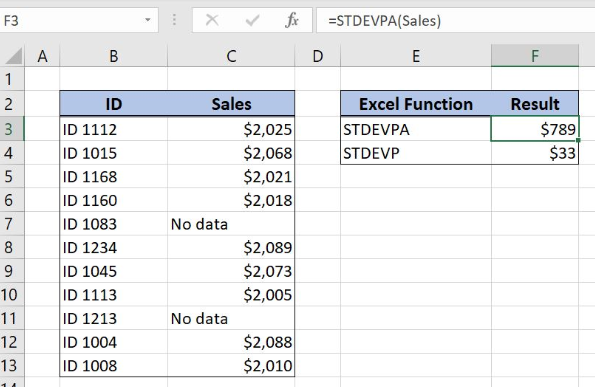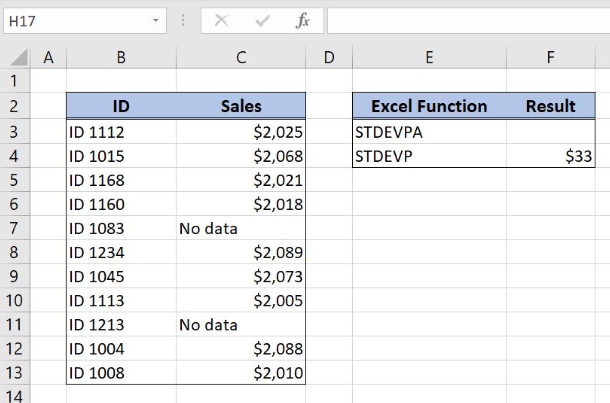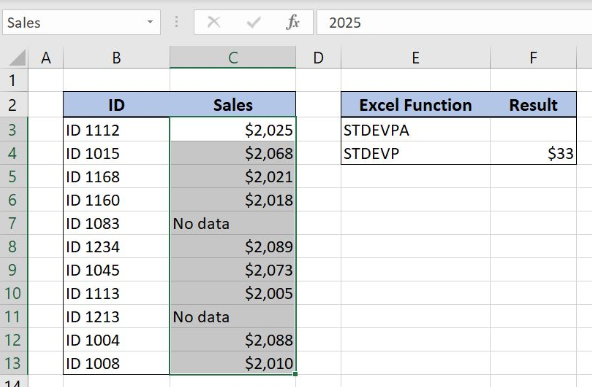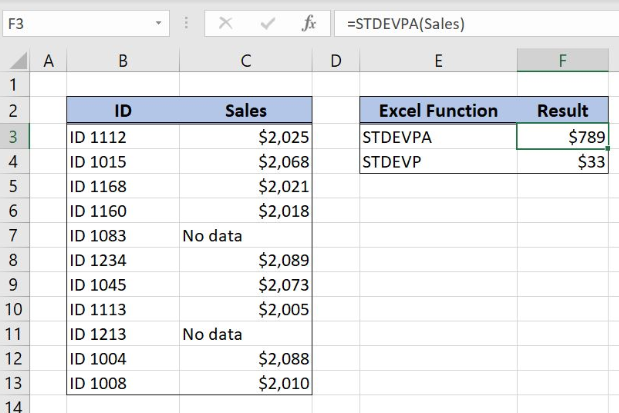Get instant live expert help with Excel or Google Sheets“My Excelchat expert helped me in less than 20 minutes, saving me what would have been 5 hours of work!”

#### Post your problem and you'll get expert help in seconds

Your message must be at least 40 characters
Our professional experts are available now. Your privacy is guaranteed.

# Excel STDEVPA Function

Excel allows us to calculate the standard deviation of the population with Excel STDEVPA Function. Comparing to STDEVP function, STDEVPA function takes into account logical and text values in the calculation. The text is interpreted as 0, TRUE as 1 and FALSE as 0. This step by step tutorial will assist all levels of Excel users in getting the standard deviation of the defined data set including text and logical values.Figure 1. Calculate the standard deviation of an entire population with STDEVPA function

## Syntax of the STDEVPA Function

`=STDEVP(value1, [value2], ...)`

The parameters of the STDEVP function are:

• value1, [value2] – the values in the data range for calculating the standard deviation for the population

## Setting up Our Data for STDEVPA Function

Our first table consists of 2 columns: “ID” (column B) and “Sales” (column C). The second one has the value of standard deviation calculated with STDEVP function and with the result placed in the cell F4. The idea is to get the standard deviation of sales data based on population with STDEVPA function and to place the result in the cell F3.Figure 2. Table structure for STDEVPA Function

## Get Standard Deviation Using STDEVPA Function

We want to get the standard deviation of the data in the “Sales” column. In order to better explain the formula, we will create a named range Sales for cell range B3:B13.

To create a named range we should follow the steps:

• Select the cell range that should be named
• Click on the name box in Excel
• Write the name for the cell range and press enterFigure 3. Creating a named range Sales for column “Sales”

The formula for getting the standard deviation for sales data is:

`=STDEVPA(Sales)`

The value1 in the STDEVPA function is the named range Sales.

To apply the STDEVPA function we need to follow these steps:

• Select cell F3 and click on it
• Insert the formula: `=STDEVPA(Sales)`
• Press enterFigure 4. Get the standard deviation of the population including text and logicals

STDEVPA function calculates the standard deviation of the population. This function evaluates logical values and text in the calculation of the standard deviation. In our example text is interpreted as zero and the formula result in the cell F3 is \$789. This result means that sales values differ \$789 from the mean of the sales data range.

Most of the time, the problem you will need to solve will be more complex than a simple application of a formula or function. If you want to save hours of research and frustration, try our live Excelchat service! Our Excel Experts are available 24/7 to answer any Excel question you may have. We guarantee a connection within 30 seconds and a customized solution within 20 minutes.